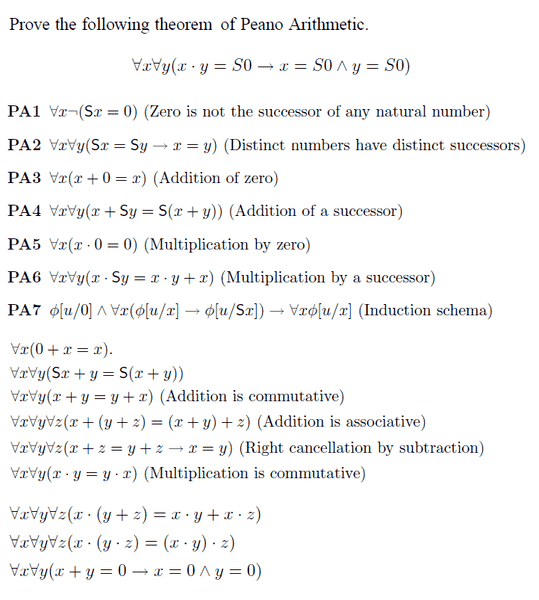# [LOGIC] Proof by Induction in Peano Arithmetic

I have to do the following using these axioms PA1-7, the others below it are previously proved results I can use too.

[Sa] means the successor of a.Base Case: y = S0

x.S0 = S0

→ x.0 + x = S0

→ 0 + x = S0

→ x = S0 & y=S0

Now the induction step is usually y=a to y=Sa, however this does not work here, I assume I need to take a new y and it's successor to proceed. Would anyone know how to proceed and which y to take?

Thanks

jgens
Gold Member
Now the induction step is usually y=a to y=Sa, however this does not work here, I assume I need to take a new y and it's successor to proceed. Would anyone know how to proceed and which y to take?

Why are you inducting here? You usually only use induction when you are trying to show that something is true for all natural numbers. So while your base case holds, any other case you try will fail.

How formally does this proof have to be done. If you are using the Peano axioms, then presumably you are taking a course in mathematical logic or something of the like, so it it alright to write the proof out in ordinary mathematical language?

Yeah we are advised to do this proof 'mathematically' rather than 'logically' (i.e not by natural deductions using rules of inference)

Here is an example proof, all the others have been done via the induction schema so I assumed this one was to be done that same way tooIf not then I have no idea how to do it without induction for those axioms

I agree with what you're saying, it doesn't make sense that this holds for any other case other than S0, but then how do I show this?

Unless I derive some contradiction using PA1, and hence I can derive anything from that contradiction..

Thanks